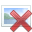# Fractioned electrons, what do they mean?

• Raul Trejo

## Homework Statement

Excess electrons are placed on a small lead sphere with mass 8.00g so that it's net charge is -3.20x10^-9C (a) Find the number of excess electrons on the sphere. (b) how many excess electrons are there per lead atom? The atomic number of lead is 82, and its atomic mass is 207g/mol.

## Homework Equations

We have -3.20x10^-9C . All charge is "quantized" meaning it is an integer multiple of the charge "e" (-1.602x10^-19C/electron). To obtain the amount of "excess" (Neutrally charged atoms have the same amount of protons and electrons, so a negatively charged atom has more electrons, the charge is due to theses extra electrons) we divide our charge Q by e. Electrons= (-3.20x10^-9C)/(-1.602x10^-19C/electron)=1.997x10^10 which we round to 2x10^10 electrons. We then obtain the number of moles the lead ball has, multiply it by avodagro's number to obtain the amount of atoms, and then divide the amount of electrons by the amount of atoms.And we end up with 8.594x10^-13electrons/atom.[/B]

## The Attempt at a Solution

I can't get my head around the fact that 8.59x10-13 electrons is not an integer multiple of 1 electron. What does it even mean to have https://www4c.wolframalpha.com/Calculate/MSP/MSP157620gi5hegf6iihfdf00005if81hhidie84ia2?MSPStoreType=image/gif&s=59 [Broken] extra electrons per atom? My understanding is that electrons are moved(transfered from one atom to the next) in integers, you can't really divide an electron to have 8.59x10^-13 of it. What does this actually mean?

Last edited by a moderator:

## Homework Statement

Excess electrons are placed on a small lead sphere with mass 8.00g so that it's net charge is -3.20x10^-19C (a) Find the number of excess electrons on the sphere. (b) how many excess electrons are there per lead atom? The atomic number of lead is 82, and its atomic mass is 207g/mol.

## The Attempt at a Solution

The problem I'm having isn't numerical in sense. The answer to the part a is 2x10^10 electrons, but the answer to part (B) is 8.59x10^-13 electrons/atom. I can't get my head around the fact that 8.59x10-13 electrons is not an integer multiple of 1 electron. What does it even mean to have https://www4c.wolframalpha.com/Calculate/MSP/MSP157620gi5hegf6iihfdf00005if81hhidie84ia2?MSPStoreType=image/gif&s=59 [Broken] extra electrons per atom? My understanding is that electrons are moved(transfered from one atom to the next) in integers, you can't really divide an electron to have 8.59x10^-13 of it. What does this actually mean?
It's not clear how you got your answer for (a). There is a definite electric charge per electron.

Can you post all of your calculations?

Last edited by a moderator:
It's not clear how you got your answer for (a). There is a definite electric charge per electron.

Can you post all of your calculations?
Note: I just realized I put -3.20x10^-19C, it's actually 10^-9.
We have -3.20x10^-9C . All charge is "quantized" meaning it is an integer multiple of the charge "e" (-1.602x10^-19C/electron). To obtain the amount of "excess" (Neutrally charged atoms have the same amount of protons and electrons, so a negatively charged atom has more electrons, the charge is due to theses extra electrons) we divide our charge Q by e. Electrons= (-3.20x10^-9C)/(-1.602x10^-19C/electron)=1.997x10^10 which we round to 2x10^10 electrons. We then obtain the number of moles the lead ball has, multiply it by avodagro's number to obtain the amount of atoms, and then divide the amount of electrons by the amount of atoms.And we end up with 8.594x10^-13electrons/atom. The answer is correct, I've checked it (Sears and Zemansky's University Physics with Modern Physics, problem 21.1). The question is, what does the fraction electrons really mean? What does it mean to have https://www4c.wolframalpha.com/Calculate/MSP/MSP157620gi5hegf6iihfdf00005if81hhidie84ia2?MSPStoreType=image/gif&s=59 [Broken] electrons extra per atom? because you can't add only a fraction of an electron to an atom.

Last edited by a moderator:
Note: I just realized I put -3.20x10^-19C, it's actually 10^-9.
We have -3.20x10^-9C . All charge is "quantized" meaning it is an integer multiple of the charge "e" (-1.602x10^-19C/electron). To obtain the amount of "excess" (Neutrally charged atoms have the same amount of protons and electrons, so a negatively charged atom has more electrons, the charge is due to theses extra electrons) we divide our charge Q by e. Electrons= (-3.20x10^-9C)/(-1.602x10^-19C/electron)=1.997x10^10 which we round to 2x10^10 electrons. We then obtain the number of moles the lead ball has, multiply it by avodagro's number to obtain the amount of atoms, and then divide the amount of electrons by the amount of atoms.And we end up with 8.594x10^-13electrons/atom. The answer is correct, I've checked it (Sears and Zemansky's University Physics with Modern Physics, problem 21.1). The question is, what does the fraction electrons really mean? What does it mean to have https://www4c.wolframalpha.com/Calculate/MSP/MSP157620gi5hegf6iihfdf00005if81hhidie84ia2?MSPStoreType=image/gif&s=59 [Broken] electrons extra per atom? because you can't add only a fraction of an electron to an atom.

You've got so many excess electrons and so many atoms on which to distribute these electrons. Having a fraction of an electron doesn't really mean anything, IMO.

Last edited by a moderator:
What does it mean to haveelectrons extra per atom?
If you eat an apple each day, how many apples per second do you eat? It is just expressing an average.